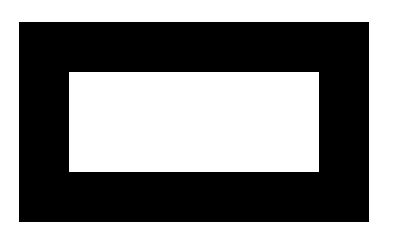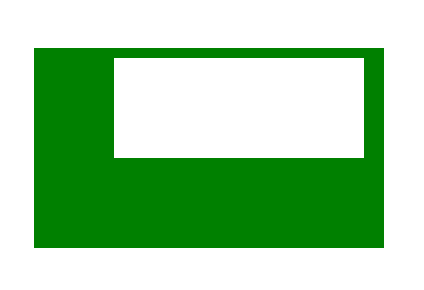# HTML canvas clearRect() Method

The clearRect() method is used to clear the specified pixels within a given rectangle. Syntax:

`context.clearRect( x, y, width, height )`

Parameters:

• x: It stores the x-coordinate of the top-left corner of the rectangle.
• y: It stores the y-coordinate of the top-left corner of the rectangle.
• width: It stores the width in pixel.
• height: It stores the height in pixel.

Example-1:

## html

 ` ` `<``html``> ` ` `  `<``head``> ` `    ``<``title``> ` `        ``HTML canvas clearRect() Method ` `    `` ` ` ` ` `  `<``body``> ` `    ``<``canvas` `id``=``"GFG"` `            ``width``=``"500"` `            ``height``=``"300"``> ` `  `` ` ` `  `    ``<``script``> ` `        ``var x = document.getElementById("GFG"); ` `        ``var context = x.getContext("2d"); ` `        ``context.fillRect(50, 50, 350, 200); ` `        ``context.clearRect(100, 100, 250, 100); ` `        ``context.stroke(); ` `    `` ` ` ` ` `  ` `

Output:Example-2:

## html

 ` ` `<``html``> ` ` `  `<``head``> ` `    ``<``title``> ` `        ``HTML canvas clearRect() Method ` `    `` ` ` ` ` `  `<``body``> ` `    ``<``canvas` `id``=``"GFG"` `            ``width``=``"500"` `            ``height``=``"300"``> ` `  `` ` ` `  `    ``<``script``> ` `        ``var x = document.getElementById("GFG"); ` `        ``var context = x.getContext("2d"); ` `        ``context.fillStyle = "green"; ` `        ``context.fillRect(50, 50, 350, 200); ` `        ``context.clearRect(130, 60, 250, 100); ` `        ``context.stroke(); ` `    `` ` ` `  ` ` ` `  ` `

Output:Supported Browsers: